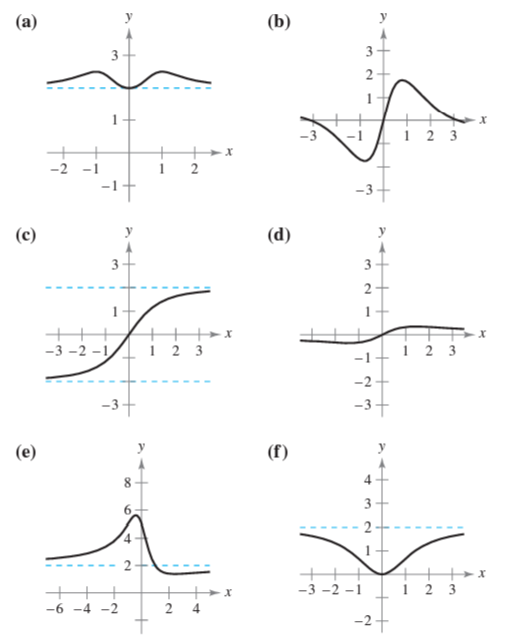×
Get Full Access to Calculus: Early Transcendental Functions - 6 Edition - Chapter 4.5 - Problem 2
Get Full Access to Calculus: Early Transcendental Functions - 6 Edition - Chapter 4.5 - Problem 2

×

# ?Matching In Exercises 1-6, match the function with one of the graphs [(a), (b), (c), (d), (e), or (f)] using horizontal asymptotes as an aid.ISBN: 9781285774770 141

## Solution for problem 2 Chapter 4.5

Calculus: Early Transcendental Functions | 6th Edition

• Textbook Solutions
• 2901 Step-by-step solutions solved by professors and subject experts
• Get 24/7 help from StudySoup virtual teaching assistantsCalculus: Early Transcendental Functions | 6th Edition

4 5 1 245 Reviews
21
3
Problem 2

Matching In Exercises 1-6, match the function with one of the graphs [(a), (b), (c), (d), (e), or (f)] using horizontal asymptotes as an aid.$$f(x)=\frac{2 x}{\sqrt{x^{2}+2}}$$

Text Transcription:

f(x)=2x/sqrt x^2+2

Step-by-Step Solution:

Step 1 of 5) The point of inflection is as shown in Figure 6.21. In Example 4, the point of inflection occurs at This is true for any logistic growth curve for which the solution starts below the carrying capacity (see Exercise 37).

Step 2 of 2

## Discover and learn what students are asking

Statistics: Informed Decisions Using Data : Comparing Three or More Means (One-Way Analysis of Variance)
?True or False: The F-test statistic is F0 = MST/MSE.

Statistics: Informed Decisions Using Data : Inference on the Least-Squares Regression Model and Multiple Regression
?Crickets make a chirping noise by sliding their wings rapidly over each other. Perhaps you have noticed that the number of chirps seems to increase wi

Unlock Textbook Solution